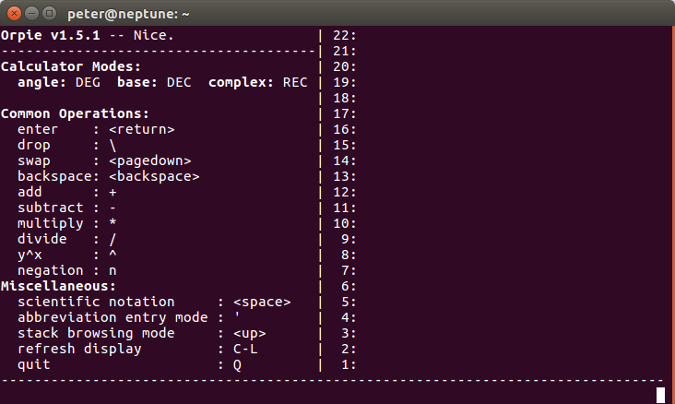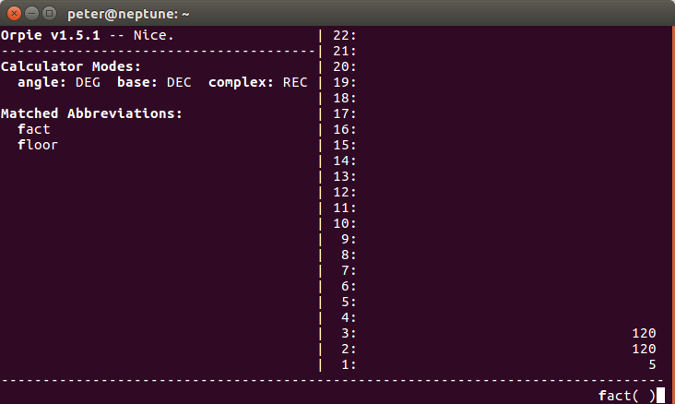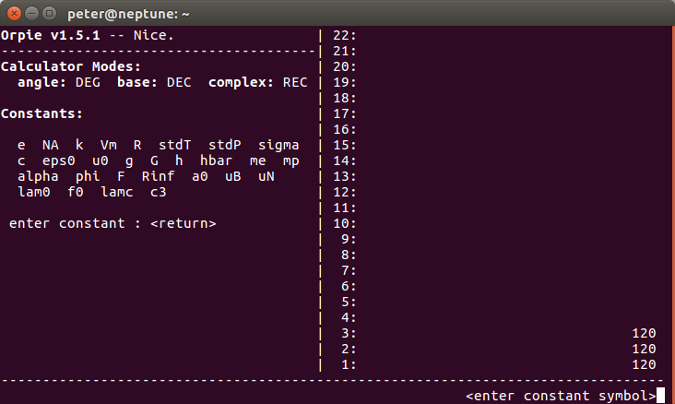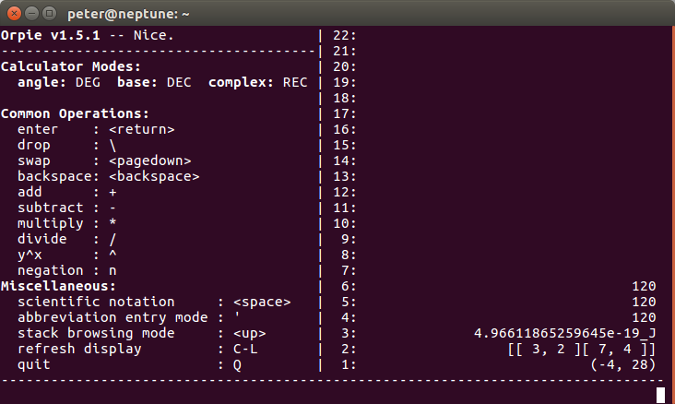# Orpie: A command-line reverse Polish notation calculator

## Orpie is a scientific calculator that functions much like early, well-loved HP calculators.Image credits :

## Subscribe now

Get the highlights in your inbox every week.

Orpie is a text-mode reverse Polish notation (RPN) calculator for the Linux console. It works very much like the early, well-loved Hewlett-Packard calculators.

## Installing Orpie

RPM and DEB packages are available for most distributions, so installation is just a matter of using either:

``\$ sudo apt install orpie``

or

``\$ sudo yum install orpie``

Orpie has a comprehensive man page; new users may want to have it open in another terminal window as they get started. Orpie can be customized for each user by editing the ~/.orpierc configuration file. The orpierc(5) man page describes the contents of this file, and /etc/orpierc describes the default configuration.

## Starting up

Start Orpie by typing orpie at the command line. The main screen shows context-sensitive help on the left and the stack on the right. The cursor, where you enter numbers you want to calculate, is at the bottom-right corner.

## orpie_start.png## Example calculation

For a simple example, let's calculate the factorial of 5 (2 * 3 * 4 * 5). First the long way:

Keys Result
2 <enter> Push 2 onto the stack
3 <enter> Push 3 onto the stack
* Multiply to get 6
4 <enter> Push 4 onto the stack
* Multiply to get 24
5 <enter> Push 5 onto the stack
* Multiply to get 120

Note that the multiplication happens as soon as you type *. If you hit <enter> after *, Orpie will duplicate the value at position 1 on the stack. (If this happens, you can drop the duplicate with \.)

Equivalent sequences are:

Keys Result
2 <enter> 3 * 4 * 5 * Faster!
2 <enter> 3 <enter> 4 <enter> 5 * * * Same result
5 <enter> ' fact <enter> Fastest: Use the built-in function

Observe that when you enter ', the left pane changes to show matching functions as you type. In the example above, typing fa is enough to get the fact function. Orpie offers many functions—experiment by typing ' and a few letters to see what's available.

## orpie_functions.pngNote that each operation replaces one or more values on the stack. If you want to store the value at position 1 in the stack, key in (for example) @factot<enter> and S'. To retrieve the value, key in (for example) @factot<enter> then ; (if you want to see it; otherwise just leave @factot as the value for the next calculation).

## Constants and units

Orpie understands units and predefines many useful scientific constants. For example, to calculate the energy in a blue light photon at 400nm, calculate E=hc/(400nm). The key sequences are:

Keys Result
C c <enter> Get the speed of light in m/s
C h <enter> Get Planck's constant in Js
* Calculate h*c
400 <space> 9 n _ m Input 4 _ 10^-9 m
/ Do the division and get the result: 4.966 _ 10^-19 J

Like choosing functions after typing ', typing C shows matching constants based on what you type.

## orpie_constants.png## Matrices

Orpie can also do operations with matrices. For example, to multiply two 2x2 matrices:

Keys Result
[ 1 , 2 [ 3 , 4 <enter> Stack contains the matrix [[ 1, 2 ][ 3, 4 ]]
[ 1 , 0 [ 1 , 1 <enter> Push the multiplier matrix onto the stack
* The result is: [[ 3, 2 ][ 7, 4 ]]

Note that the ] characters are automatically inserted—entering [ starts a new row.

## Complex numbers

Orpie can also calculate with complex numbers. They can be entered or displayed in either polar or rectangular form. You can toggle between the polar and rectangular display using the p key, and between degrees and radians using the r key. For example, to multiply 3 + 4i by 4 + 4i:

Keys Result
( 3 , 4 <enter> The stack contains (3, 4)
( 4 , 4 <enter> Push (4, 4)
* Get the result: (-4, 28)

Note that as you go, the results are kept on the stack so you can observe intermediate results in a lengthy calculation.

## orpie_final.png## Quitting Orpie

You can exit from Orpie by typing Q. Your state is saved, so the next time you start Orpie, you'll find the stack as you left it.